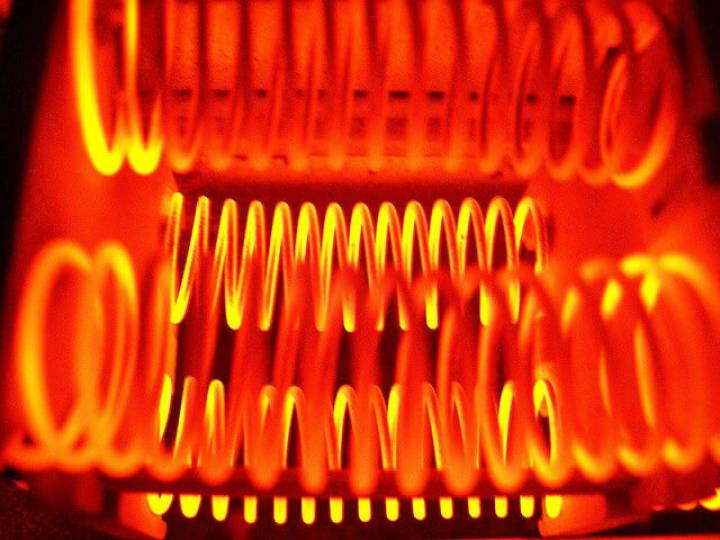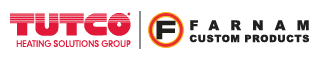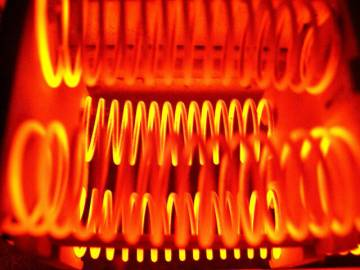Engineer Talk

# Heater Coil DesignBasic Specifications

Wattage
In the design of a custom open coil heating element, several factors need to be considered when selecting the optimum coil(s) for an application. First, the watts, volts, and resistance must be determined for each coil in the heater.  Typically, the line voltage is specified by the customer and/or end-user. The total wattage is calculated using the amount of airflow (SCFM) and the temperature rise (∆T) desired.  (W=SCFM∙∆T /3 ) The wattage of each coil is the total wattage divided by the number of coils in the circuit.  Most heaters use identical coils throughout the circuit to ensure that the load is balanced.

Voltage
The voltage across each coil is determined by the circuit used.  A single-phase heater will ‘see’ full line voltage unless some of the coils are in series.  A three-phase heater is the same if it is wired as a delta circuit.  A wye (star) circuit will ‘see’ only 57.7% of the full line voltage on each leg.  If there are two coils in series on the legs, the voltage each ‘sees’ will be one half of the voltage on the leg.

Resistance
The resistance is calculated using Ohm’s Law (R = V2/W).  The result is Rhot. But Rhot is the resistance of the wire when it’s hot. Resistance increases with temperature, so compensation must be made to get Rcold.  Rcold is the resistance when at room temperature. The various alloys have different temperature factors (T.F.) from hot to cold. We generally use a T.F. of either -3% or -5% to figure Rcold from Rhot depending on the alloy.  For example, a 1000W 120V heater would have a Rhot of 14.4Ω.  (120V2/1000W=14.4Ω)  Using -5% T.F. will result in Rcold of 13.68Ω.  (14.4Ω x .95 = 13.68Ω)

Detailed Specifications and Coil Geometry

Alloy
The next thing to be determined is which alloy to use for the resistance wire.  Each alloy has different properties that need to be considered for each application.  Some alloys are stronger at high temperatures, some resist oxidation better at high temperatures,  and some have non-magnetic properties.

Dimensions
The coil designer must now fit the coil to the heater frame he will use.  This allows him to calculate how long each coil must be, and this is called the Stretch Length (S.L.).  A coil usually exits the coiling machine with the coil completely ‘closed’, with each wrap of the coiled wire against one another.  When the coil is stretched it will open up so that the wraps are not touching.  The amount it opens is referred to as the Stretch Ratio (S.R. = Stretch Length/Closed Length).  Stretch Ratio is the geometric pitch from one coil wrap to the adjacent one, expressed in units of the wire diameter.  A closed coil (not stretched) has an S.R. of 1.00.  An S.R. of 2.00 has a Space Between Turns (S.B.T.) equal to one diameter of the wire.  A properly designed coil will have its stretch ratio between the minimum and maximum values necessary for that type of heater, usually between 2.5 and 4.0.  This allows the air to circulate around the wire for better heat transfer.

The I.D. of the ‘donut’ bushings used to support the coil, in a duct heater, for instance, determines the maximum O.D. for the coil.  The O.D. is controlled by specifying a suitable arbor on which to wind the coil.

Wire Gauge
The wire gauge selected has even more properties to consider, such as “the finer the wire, the higher the resistance”, measured in Ω/ft.  Using a finer wire increases the watt density (W/In2) for a given O.D. The arbor to wire ratio (A/W) will get larger with a finer wire.  If the A/W ratio is too large, the coil will be too flimsy and will sag.  If it’s too small the coil will be too stiff to stretch, and will also be more difficult to wind onto the heater frame.

We have produced thousands of open coil heater designs used in a wide range of applications and industries around the world. Contact us about your next project and see what Tutco-Farnam can manufacture for you.

Tutco-Farnam Custom ProductsCalculators
Calculators +